Home | | Physics 12th Std | Motional emf from FaradayŌĆÖs law and Energy conservation

# Motional emf from FaradayŌĆÖs law and Energy conservation

Physics : Electromagnetic Induction: Motional emf from FaradayŌĆÖs law and Energy conservation

Motional emf from FaradayŌĆÖs law and Energy conservation

Let us consider a rectangular conducting loop of width l in a uniform magnetic fieldwhich is perpendicular to the plane of the loop and is directed inwards. A part of the loop is in the magnetic field while the remaining part is outside the field as shown in Figure 4.10.When the loop is pulled with a constant velocityto the right, the area of the portion of the loop within the magnetic field will decrease. Thus, the flux linked with the loop will also decrease. According to FaradayŌĆÖs law, an electric current is induced in the loop which flows in a direction so as to oppose the pull of the loop.

Let x be the length of the loop which is still within the magnetic field, then its area is lx . The magnetic flux linked with the loop isAs this magnetic flux decreases due to the movement of the loop, the magnitude of the induced emf is given byHere,  both  B  and  l  are  constants. Therefore,where v = dx/dt is the velocity of the loop.This emf is known as motional emf since it is produced due to the movement of the loop in the magnetic field.

From LenzŌĆÖs law, it is found that the induced current flows in clockwise direction. If R is the resistance of the loop, then the induced current is given by## Energy conservation

In order to move the loop with a constant velocity, a constant force that is equal and opposite to the magnetic force, must be applied. Therefore, mechanical work is done to move the loop. Then the rate of doing work or power isNow, let us find the magnetic force acting on the loop due to its movement in the magnetic field. Let three deflecting forces1 ,2 and3 be acting on the three segments of the loop as shown in Figure 4.10. The general equation of such a deflecting force is given byForces2 and3 are equal in magnitude and opposite in direction and cancel each other. Therefore, the force1 alone acts on the left segment of the loop in a direction shown in Figure 4.10 and is given by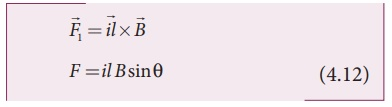Here ╬Ė is the angle betweenand the length vector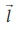for the left segment and is 90┬║

Ōł┤ F1 = il Bsin90┬║ =il B    since sin90┬║ = 1

The applied forcemust be equal toin order to just move the loop with a 1 constant velocity(sinceand1 are in opposite direction)

Considering only the magnitudes,

F = F1 = i l B

Substituting for i from equation (4.10)From equation (4.11), the rate at which the mechanical work is done to pull the loop from the magnetic field or power is given byWhen the induced current flows in the loop, Joule heating takes place. The rate at which thermal energy is dissipated in the loop or power dissipated isThis equation is exactly same as the equation (4.13). Thus the mechanical work done in moving the loop appears as thermal energy in the loop.

EXAMPLE 4.8

A conducting rod of length 0.5 m falls freely from the top of a building of height 7.2 m at a place in Chennai where the horizontal component of EarthŌĆÖs magnetic field is 40378.7 nT. If the length of the rod is perpendicular to EarthŌĆÖs horizontal magnetic field, find the emf induced across the conductor when the rod is about to touch the ground. [Take g = 10 m s-2]

Solution

l = 0.5 m; h = 7.2 m; u = 0 m s-1;

g = 10 m s-2; BH = 40378.7 nT

The final velocity of the rod isInduced emf when the rod is about to touch the ground, ╬Ą = BH lv

= 40, 378.7 ├Ś10ŌłÆ9 ├Ś 0.5 ├Ś12

=242 .27 ├Ś10ŌłÆ6V

=242 .27┬ĄV

EXAMPLE 4.9

A copper rod of length l rotates about one of its ends with an angular velocity Žē in a magnetic field B as shown in the figure. The plane of rotation is perpendicular to the field. Find the emf induced between the two ends of the rod.

Solution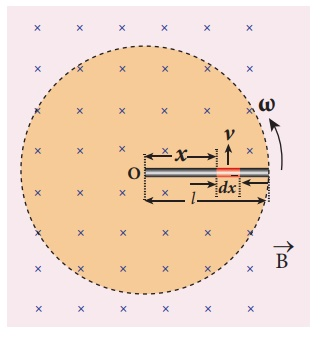Consider a small element of length dx at a distance x from the centre of the circle described by the rod. As this element moves perpendicular to the field with a linear velocity v = xŽē , the emf developed in the element dx is

d ╬Ą = Bvdx = B( xŽē)dx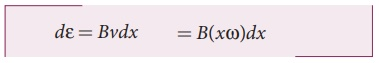This rod is made up of many such elements, moving perpendicular to the field. The emf developed across two ends is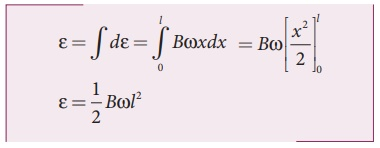Tags : Definition, Explanation, Solved Example Problems | Electromagnetic Induction , 12th Physics : Electromagnetic Induction and Alternating Current
Study Material, Lecturing Notes, Assignment, Reference, Wiki description explanation, brief detail
12th Physics : Electromagnetic Induction and Alternating Current : Motional emf from FaradayŌĆÖs law and Energy conservation | Definition, Explanation, Solved Example Problems | Electromagnetic Induction